Conge 精进

# ML4T笔记 | 03-07 Dyna

2019-03-31

## 1 - Overview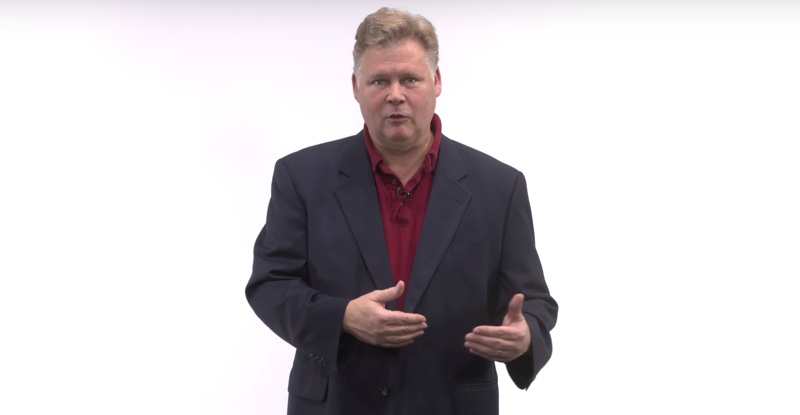Q-learning is expensive because it takes many experienced tuples to converge. Creating experienced tuples means taking a real step to execute a trade, in order to gather information.

To address this problem, Rich Sutton invented Dyna.

• Dyna models T (the transition matrix) and R (the reward matrix).
• after each real interaction with the world, we hallucinate many additional interactions, usually a few hundred that are used then to update the queue table.

Time: 00:00:37

## 2 - Dyna-Q Big Picture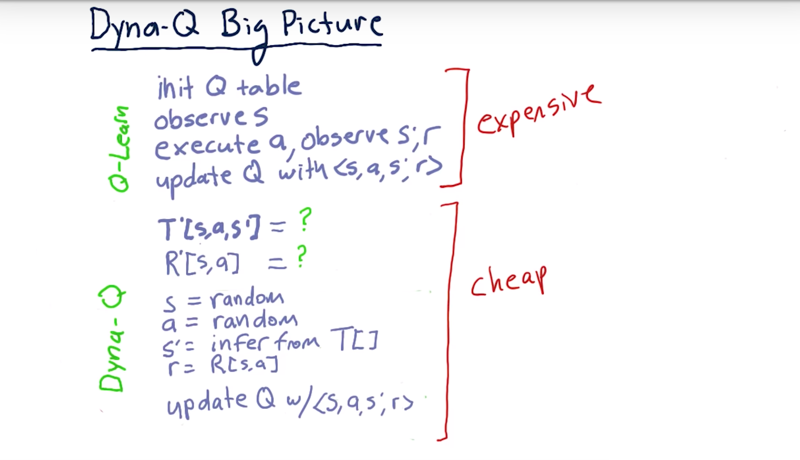Q learning is model-free meaning that it does not rely on T or R.

• Q learning does not know T or R.
• T = transition matrix and R = reward function,

Dyna-Q is an algorithm developed by Rich Sutton intended to speed up learning or model convergence for Q learning.

Dyna is a blend of model-free and model-based methods.

1. first consider plain old Q learning, initialize our Q table and then we begin iterating, observe s, take action a, and then observe our new state s' and reward r, and then update Q table with this experience tubal and repeat.
2. when we augment Q learning with Dyna-Q, we had three new components, the first is that we add some “logic that enables us to learn models of T and R”, then we hallucinate an experience.
• hallucinate these experiences, update our queue table and repeat this many times, maybe hundreds of times.
• This operation is very cheap compared to interacting with the real world.
• After we’ve iterated enough times down here maybe 100 maybe 200 times then we return and resume our interaction with the real world.

### Let’s look at each of these components in a little more detail now.

When updating our model we want to do is find new values for T and R.

The point where we update our model includes T, our reward function R.

• T is the probability that if we are in state s and we take action a will end up in s prime.
• r is our expected reward if we are in state s and we take action a.

### how we hallucinate an experience.

1. randomly select an s,
2. randomly select an a, so our state and action are chosen totally at random.
3. infer our new state s' by looking at T.
4. we infer a reward r by looking at big R or R table.

Now we have a complete experience tuple to update our Q table using that.

So, the Q table update is our final step and this is really all there is to die in a queue.

Time: 00:04:14

## 3 - Learning T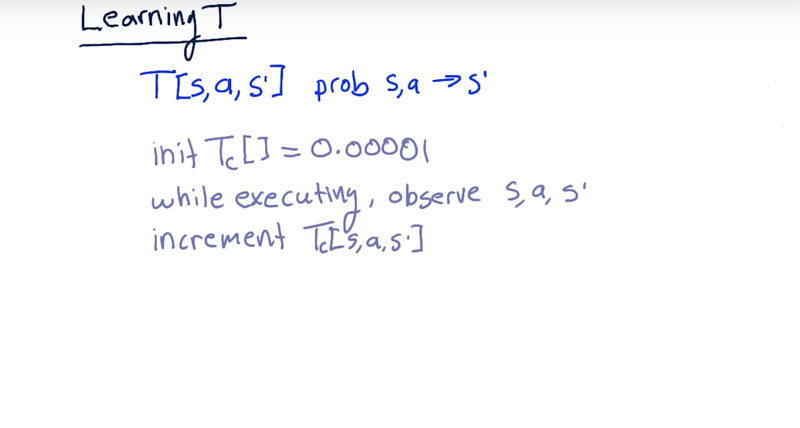Note the methods are Balch version, might not be Rich Sutton version.

Learning T.

T[s,a,s’] represents the probability that if we are in the state s, take action a we will end up in state s'.

To learn a model of T: just observe how these transitions occur.

• T count (TC): when we have experience with the real world, we’ll get back on <s,a,s’> and we’ll just count how many times did it happen.
• initialize all of our T count values to be a very, very small number to avoid number divided by zero.
• Each time we interact with the real world in Q-learning, we observe <s,a,r,s’>, then we just increment that location in our T-count matrix.

Time: 00:01:34

## 4 - How to Evaluate T

$T[s,a,s’] = \frac{T_c[s,a,s’]}{\sum\limits_{i}T_c[s,a,i]}$

Time: 00:01:03

## 6 - Learning R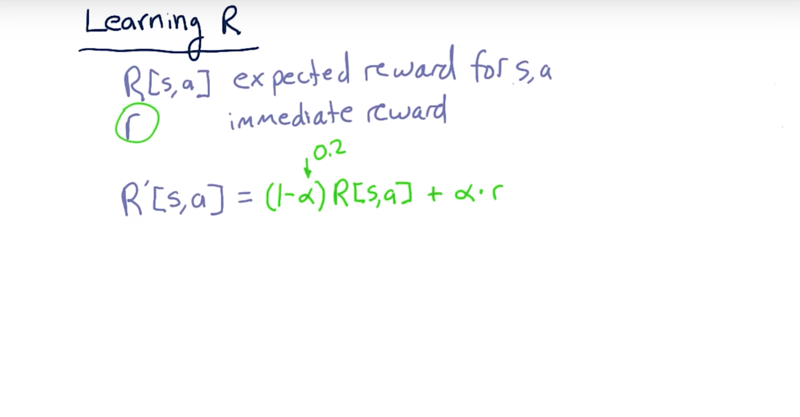The last step here is how do we learn a model for R?

• When we execute an action a in state s, we get an immediate reward, r.

• R[s, a] are expected reward if we’re in state s and we execute action a.
• R is our model, r is what we get in an experience tuple.
• update R model every time we have a real experience.
• $R’[s,a] = (1 - \alpha) R[s,a] + \alpha \times r$.
• r is the new estimate. That’s a simple way to build a model of R from observations of interactions with the real world.

Time: 00:01:39

## 7 - Dyna Q Recap

how Dyna-Q works.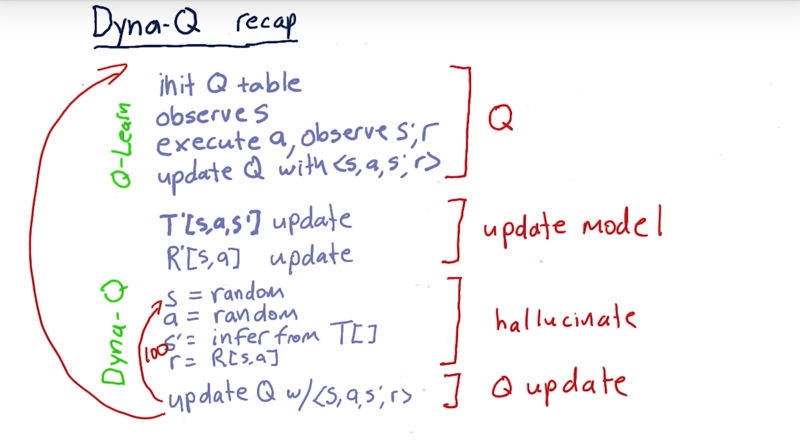Dyna-Q adds three new components based on regular Q-Learning

1. update models of T and R,
2. then we hallucinate an experience.
3. update our Q table.

We can repeat 2-3 many times. ~100 or 200 here, then return back up to the top and continue our interaction with the real world.

• The reason Dyna-Q is useful: use cheap hallucinations to replace real-world interaction (which is expensive)

• And when we iterate doing many of them, we update our Q table much more quickly.

Time: 00:00:57

Total Time: 00:10:41

## Summary

The Dyna architecture consists of a combination of:

• direct reinforcement learning from real experience tuples gathered by acting in an environment,
• updating an internal model of the environment, and,
• using the model to simulate experiences.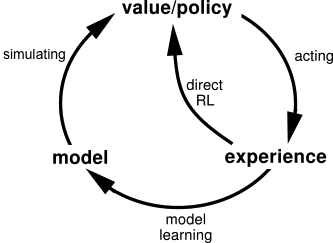Sutton and Barto. Reinforcement Learning: An Introduction. MIT Press, Cambridge, MA, 1998. [web]

## Resources

• Richard S. Sutton. Integrated architectures for learning, planning, and reacting based on approximating dynamic programming. In Proceedings of the Seventh International Conference on Machine Learning, Austin, TX, 1990. [pdf]

• Sutton and Barto. Reinforcement Learning: An Introduction. MIT Press, Cambridge, MA, 1998. [web]

• RL course by David Silver (videos, slides)

• Lecture 8: Integrating Learning and Planning [pdf]
2019-03-31 初稿
`